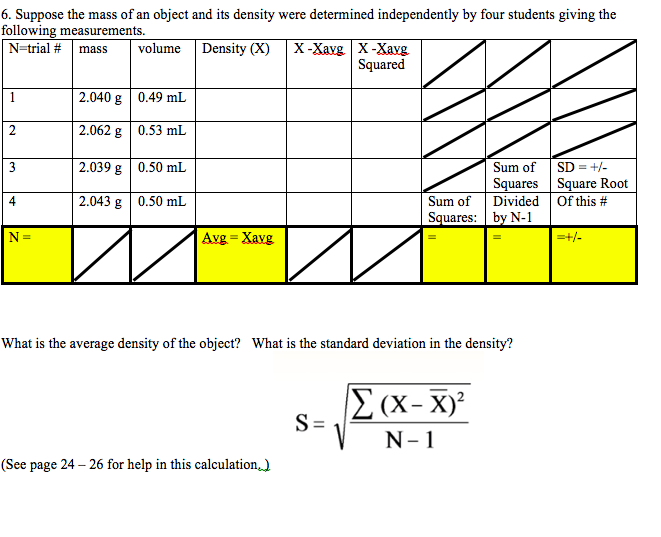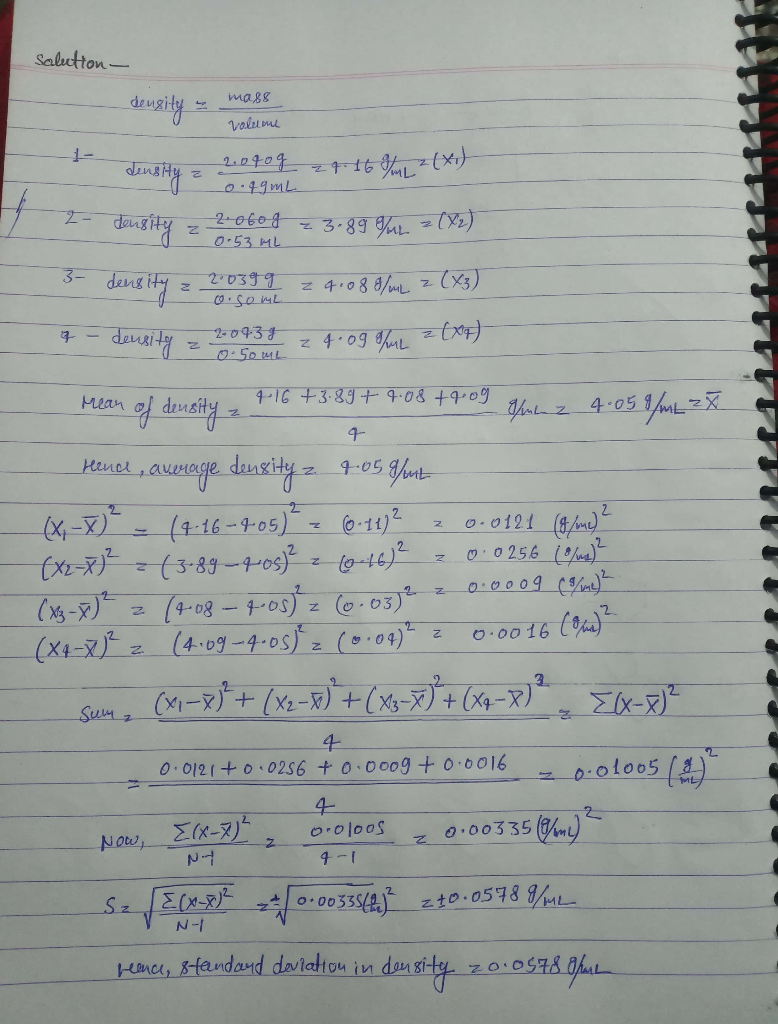# 6. Suppose the mass of an object and its density were determined independently by four students...6. Suppose the mass of an object and its density were determined independently by four students giving the following measurements. N=trial # mass volume Density (X) X-Xavg X-Savg Squared 2.040 g 0.49 mL 2.062 g 0.53 mL 2.039 g 0.50 mL 2.043 g 0.50 mL Sum of SD = + - Squares Square Root Sum of Divided Of this # Squares: by N-1 =+/- Avg = Xavg What is the average density of the object? What is the standard deviation in the density? (x-7)? S=1 N-1 dhe se (See page 24 - 26 for help in this calculation)

It is simple calculation based.